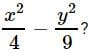## 20 Questions MCQ Test Mathematics (Maths) Class 8 | Olympiad Test: Factorisation

Description
Attempt Olympiad Test: Factorisation | 20 questions in 20 minutes | Mock test for Class 8 preparation | Free important questions MCQ to study Mathematics (Maths) Class 8 for Class 8 Exam | Download free PDF with solutions
QUESTION: 1

### What are the factors of ax+by+bx+az+ay+bz?

Solution:

ax+by+bx+az+ay+bz, rearranging we get ax+ay+az+bx+by+bz
a(x+y+z)+b(x+y+z)=(a+b)(x+y+z). Hence the factors are (a+b), (x+y+z)

QUESTION: 2

### Which of the following is one of the factors of x4+4?

Solution:

x⁴ + 4

= (x² + 2)² - 4x²

= (x² + 2)² - (2x)²

a² - b² = (a + b)(a - b)

= (x² + 2 + 2x)(x² + 2 - 2x)

QUESTION: 3

### What are the factors of x4+2x2+9?

Solution:

Given equation is x4 + 2x2 + 9
We can rewrite this as,
(x2)2 + 6x2 + 9 − 4x2
⇒ (x2 + 3)2 − (2x)2
....Since a2 + 2ab + b2 = (a+b)2
⇒ x4 + 2x2 + 9 = (x2 − 2x + 3)(x2 + 2x + 3)
....Since a− b2 = (a+b)(a−b)

QUESTION: 4

For x2+2x+5 to be a factor of x4+ px2+q, what must the respective values of p and q be?

Solution:

Let the other factor be x2+ax+b. We have (x2+2x+5)(x2+ax+b)
= x4+px2+q
x4(2+a)x3(2a+b+5)x2(5a+2b)x+5b = x4+px2+q
Comparing the coefficients of corresponding terms, we get 2a+b+5 = p  ......(1)
5b = q   ......(2)
2+a = 0 ⇒ a =−2
5a+2b = 0 ⇒ b = 5
∴ p = 2a+b+5 = 2(−2)+5+5 = 6
q = 5b = 5(5) = 25

QUESTION: 5

What are the factors of x2+xy−2xz−2yz?

Solution:

x² + xy - 2xz - 2yz

x(x + y).-2z(x + y)

(x - 2z)(x + y)

QUESTION: 6

Amrit and Pankaj expanded (x−5)2. Amrit's answer is x2−25 and Pankaj's answer is x2−10x+25. Which of the following statements is correct?

Solution:

(x−5)2 = x2−10x+25 using

(a−b)2 = a2−2ab+b2. So, Pankaj's answer is correct.

QUESTION: 7

Find the quotient when 5a2b2c2 is divided by 15abc.

Solution:
QUESTION: 8

Which of the following statements is correct?

Solution:

(a−4)(a−2) = a2−4a−2a+8 = a2−6a+8 So, the statement in option (A) is correct.

QUESTION: 9

What are the factors of x4+y4+x2y2?

Solution:

x4+y4+x2y2 =(x4+y4+2x2y2)−x2y2 =(x2+y2)2−(xy)2
=(x2+y2+xy)(x2+y2−xy)

QUESTION: 10

Choose the factors of 15x2−26x+8 from the following.

Solution:

15x2−26x+8 =15x2−20x−6x+8 =5x(3x−4)−2(3x−4) =(3x−4)(5x−2)

QUESTION: 11

How many factors does (x9−x) have?

Solution:

f(x)=x9−x
=x(x8−1)
=x[(x4)2−(1)2]
=x[(x4−1)(x4+1)]
=x(x4+1)[(x2)2−12]
=x(x4+1)(x2+1)(x2−1)
=x(x4+1)(x2+1)(x−1)(x+1)
∴ There are 5 factors of f(x)⇒(x),(x4+1),(x2+1),(x−1) and (x+1)

QUESTION: 12

Which of the following are the factors ofSolution:
QUESTION: 13

What is the coefficient of 'a' when 9a2+18a is divided by (a+2)?

Solution:
QUESTION: 14

From the following, which are the factors of a- b + ab - a?

Solution:

a² - b + ab - a = a² + ab - b - a

= ( a² + ab ) - ( b + a )

= a ( a + b) - (a + b )

= ( a + b) ( a - 1 )

QUESTION: 15

The expression (p2+7p+10) is factorized and then divided by (p+5). What is the quotient?

Solution:

p^2 + 7p + 10 / p + 5

p^2 + 5p + 2p + 10 / p+5

p ( p + 5 ) + 2(p + 5 ) / p+5

( p+5) ( p+ 2 ) / p + 5

( p+2)

QUESTION: 16

Which is the correct statement in the following?

Solution:

n(3n+2) = 3n2+2n

QUESTION: 17

If (x2+3x+5)(x2−3x+5) = m2−n2, what is the value of m?

Solution:

(x2+3x+5)(x2−3x+5) = (x2+5+3x)(x2+5−3x) = (x2+5)2−(3x)= m2−n2
∴ m = x2+5

QUESTION: 18

Divide 6p5+18p4−3p2 by 3p2.

Solution:
QUESTION: 19

Find the factors of b2−7b+12.

Solution:

b- 7b+12=b2-3b-4b+12=b(b-3)-4(b-3)=(b-3)(b-4)

QUESTION: 20

Find the factors of6 mn−4n+6−9m.

Solution:

6mn-9m-4n+6

take 3m common

3m(2n-3)-2(2n-3)

(3m-2)(2n-3)Use Code STAYHOME200 and get INR 200 additional OFF Use Coupon Code